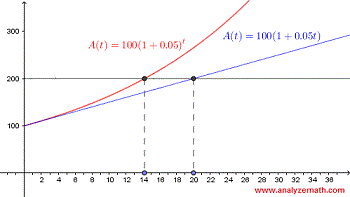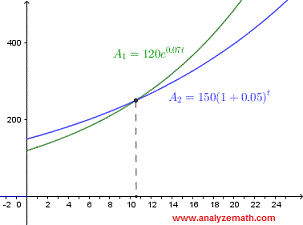### Popular Pages# Compound Interest Problems with Detailed Solutions

Compound interest problems with answers and solutions are presented.

1. A principal of $2000 is placed in a savings account at 3% per annum compounded annually. How much is in the account after one year, two years and three years? 2. What would$1000 become in a saving account at 3% per year for 3 years when the interest is not compounded (simple interest)? What would the same amount become after 3 years with the same rate but compounded annually?
3. $100 is the principal deposited in a 5% saving account not compounded (simple interest). The same amount of$100 is placed in a 5% saving account compounded annually. Find the total amount A after t years in each saving plan and graph both of them in the same system of rectangular axes. Use the graphs to approximate the time it takes each saving plan to double the initial amount.
4. If $3000 is placed in an account at 5% and is compounded quarterly for 5 years. How much is in the account at the end of 5 years? 5.$1200 is placed in an account at 4% compounded annually for 2 years. It is then withdrawn at the end of the two years and placed in another bank at the rate of 5% compounded annually for 4 years. What is the balance in the second account after the 4 years.
6. $1,200 is placed in an account at 4% compounded daily for 2 years. It is then withdrawn and placed in another bank at the rate of 5% compounded daily for 4 years. What is the balance in the second account after the 4 years. (compare with the previous problem) 7.$1200 is placed in an account at 4% compounded continuously for 2 years. It is then withdrawn and placed in another bank at the rate of 5% compounded continuously for 4 years. What is the balance in the second account after the 4 years. (compare with the two previous problem)
8. What principal you have to deposit in a 4.5% saving account compounded monthly in order to have a total of $10,000 after 8 years? 9. A principal of$120 is deposited in a 7% account and compounded continuously. At the same time a principal of $150 is deposited in a 5% account and compounded annually. How long does it take for the amounts in the two accounts to be equal? 10. A first saving account pays 5% compounded annually. A second saving account pays 5% compounded continuously. Which of the two investments is better in the long term? 11. What interest rate, compounded annually, is needed for a principal of$4,000 to increase to $4,500 in 10 year? 12. A person deposited$1,000 in a 2% account compounded continuously. In a second account, he deposited $500 in a 8% account compounded continuously. When will the total amounts in both accounts be equal? When will the total amount in the second accounts be 50% more than the total amount in the second account? 13. A bank saving account offers 4% compounded on a quarterly basis. A customer deposit$200, in this type of account, at the start of each quarter starting with the first deposit on the first of January and the fourth deposit on the first of October. What is the total amount in his account at the end of the year?
14. An amount of $1,500 is invested for 5 years at the rates of 2% for the first two years, 5% for the third year and 6% for the fourth and fifth years all compounded continuously. What is the total amount at the end of the 5 years? Solutions to the Above Questions 1. Solution When interest is compounded annually, total amount A after t years is given by: A = P(1 + r) t, where P is the initial amount (principal), r is the rate and t is time in years. 1 year: A = 2000(1 + 0.03) 1 =$2060
2 years: A = 2000(1 + 0.03) 2 = $2121.80 3 years: A = 2000(1 + 0.03) 3 =$2185.45
2. Solution
Not compounded: A = P + P(1 + r t) = 1000 + 1000(1 + 0.03 � 3) = $1090 Compounded: A = P(1 + r) t = 1000(1 + 0.03) 3 =$1092.73
Higher return when compounded.
3. Solution
Not compounded: A = P + P(1 + r t) = 100(1 + 0.05 t)
Compounded: A = P(1 + r) t = 100(1 + 0.05) t
Graphs below are those of the compounded and not compounded interests. The compounded interest doubles in about 14 years while the non compounded (simple) interest doubles in about 20 about years.4. Solution
Compounded n times a year and after t years, the total amount is given by: A = P(1 + r/n) n t
quarterly n = 4: Hence A = P(1 + r/4) 4 t = 3000(1 + 0.05/4) 4 � 5 = $3846.11 5. Solution Annual compounding First two years: A = P(1 + r) t = 1200(1 + 0.04) 2 =$1297.92
Last four years : A = P(1 + r) t = 1297.92(1 + 0.05) 4 = $1577.63 6. Solution Daily compounding (assuming 365 days per year) First two years: A = P(1 + r / 365) 365 t = 1200(1 + 0.04 / 365) 365 � 2 =$1299.94
Last four years : A = P(1 + r / 365) 365 t = 1299.94 (1 + 0.05 / 365) 365 �4 = $1587.73 Higher final balances compared to annual compounding in last problem. 7. Solution In continuous compounding, final balance after t years is given by: A = P e r t. First two years: A = P e r t = 1200 e 0.04 � 2 =$1299.94
Last four years : A = P e r t = $1299.94 e 0.05 � 4 =$1587.75
Same balances compared to daily compounding in last problem.
8. Solution
P initial balance to find and final balance A known and equal to $10,000. A = P(1 + 0.045 / 12) 12 � 8 = 10,000 P = 10,000 / ( (1 + 0.045 / 12) 12 � 8 ) =$6981.46
9. Solution
Continuous compounding: A 1 = 120 e 0.07 t
Annual compounding: A 2 = 150 (1 + 0.05)t
A 1 = A 2
120 e 0.07 t = 150 (1 + 0.05)t
Take log base e (ln) of both sides.
ln(120 e 0.07 t) = ln( 150 (1 + 0.05)t )
Use property ln(A B) = ln(A) + ln(B) to rewrite the above as:
ln(120) + ln(e 0.07 t) = ln(150) + ln ( (1 + 0.05)t )
Use properties ln A n = n ln(A), ln(e n) = n to simplify.
ln(120) + 0.07 t = ln(150) + t ln( 1+ 0.05)
Solve for t.
t (0.07 - ln( 1 + 0.05) ) = ln(150) - ln(120)
t = [ ln(150) - ln(120) ] / [ 0.07 - ln( 1 + 0.05) ] ≅ 10.5 years
Check graphically below. Graphs intersect when A 1 = A 2.10. Solution
Continuous compounding: A 1 = 100 e 0.05 t
Annual compounding: A 2 = 100 (1 + 0.05)t
From graphs below, at equal rates, the continuous compounding earns more that the annual compounding in the long term.11. Solution
P initial balance is equal to $4,000 and final balance is equal to$4,500.
A = P(1 + r) t = 4,500
4000(1 + r) 10 = 4,500
(1 + r) 10 = 4500 / 4000
Take ln of both sides.
10 ln(1 + r) = ln(4500 / 4000)
ln(1 + r) = ln(4500 / 4000) / 10
1 + r = e 0.1 ln(4500 / 4000)
r = e 0.1 ln(4500 / 4000) - 1 ≅ 0.012
12. Solution
A 1 = 1000 e 0.02 t
A 2 = 500 e 0.08 t
A 1 = A 2 gives
1000 e 0.02 t = 500 e 0.08 t
Divide both sides by 500 e 0.02 t and simplify
100 / 500 = e 0.08 t - 0.02 t
2 = e 0.06 t
Take ln of both sides.
0.06 t = ln 2
t = ln 2 / 0.06 ≅ 11.5 years
A 2 is 50% more than A 1 gives the equation:
A 2 = 1.5 A 1
500 e 0.08 t = 1.5 � 1000 e 0.02 t
e 0.08 t - 0.02 t = 3
0.06 t = ln 3
t ≅ 18.5 years
13. Solution
A = P(1 + r/n) n t
First quarter deposit, t = 1 year: A 1 = 200 (1 + 0.04 / 4) e 4 � 1 = $208.12 Second quarter deposit, t = 3/4 of 1 year : A 2 = 200 (1 + 0.04 / 4) e 4 � 3/4 =$206.06
Third quarter deposit , t = 1/2 of 1 year : A 3 = 200 (1 + 0.04 / 4) e 4 � 1/2 = $204.02 Fourth quarter deposit, t = 1/4 of 1 year : A 4 = 200 (1 + 0.04 / 4)e 4 � 1/4 =$202
Total amount = $208.12 +$206.06 + $204.02 +$202 = $820.2 14. Solution A = P e r t End of first two years: A 1 = 1500 e 0.02 � 2 End of third year: A 2 = A 1 e 0.05 � 1 End of fifth year (last two years): A 3 = A 2 e 0.06 � 2 = 1500 e 0.02 � 2 + 0.05 � 1 + 0.06 � 2 =$1850.51

High School Maths (Grades 10, 11 and 12) - Free Questions and Problems With Answers
Middle School Maths (Grades 6, 7, 8, 9) - Free Questions and Problems With Answers
Primary Maths (Grades 4 and 5) with Free Questions and Problems With Answers
Author - e-mail